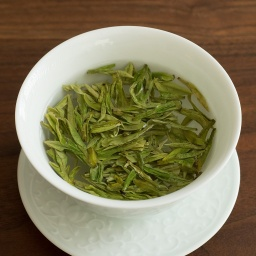# Merchant 6730

A merchant sells a blend of tea. Kilogram for 450 CZK. Another species for 900 CZK. Cheaper for 369 CZK. How much tea must buy per 5 kg of the mix?

a =  4.2373
b =  0.7627

### Step-by-step explanation:

a+b = 5
369a + 900b = 5•450

a+b = 5
369•a + 900•b = 5•450

a+b = 5
369a+900b = 2250

a = 250/59 ≈ 4.237288
b = 45/59 ≈ 0.762712

Our linear equations calculator calculates it.Did you find an error or inaccuracy? Feel free to write us. Thank you!

Tips for related online calculators
Do you have a system of equations and looking for calculator system of linear equations?